## 微分和导数的关系是什么？两者的几何意义有什么不同？为什么要定义微分 ?

• 对于导数的链式法则，$\frac{dy}{dx}=\frac{dy}{du}\frac{du}{dx}$，可以理解为$du$可以约去，所以两者相等。但假如有$F(x,y)$，$\frac{dy}{dx} = -\frac{\partial F/\partial x}{\partial F/\partial y}$，通过消去我们是否可以推出$\frac{dy}{dx}=-\frac{dy}{dx}$？
• $\int _ a^ b \frac{dy}{dx}dx\implies \int _ a^ b dy\implies y\rvert _ a^ b$，这里好像实实在在的消去了$dx$。
• $d(uv)=(u+du)(v+dv)-uv=udv+vdu+dudv$，然后说$dudv$太小了，所以忽略，得到了微分的乘法法则，$d(uv)=udv+vdu$，难道$udv$和$vdu$不小！！

1 牛顿、莱布尼兹开始的古典微积分

1.1 符号说明

• $\Delta x$：差分
• $dx$：微分
• $f'(x)$：导数，也可以写成$\frac{dy}{dx}$，所以也可以叫做微商

1.2 导数为什么出现？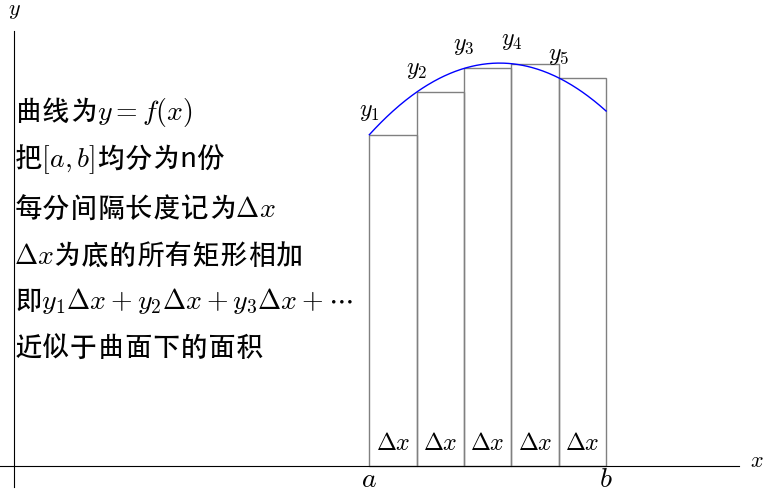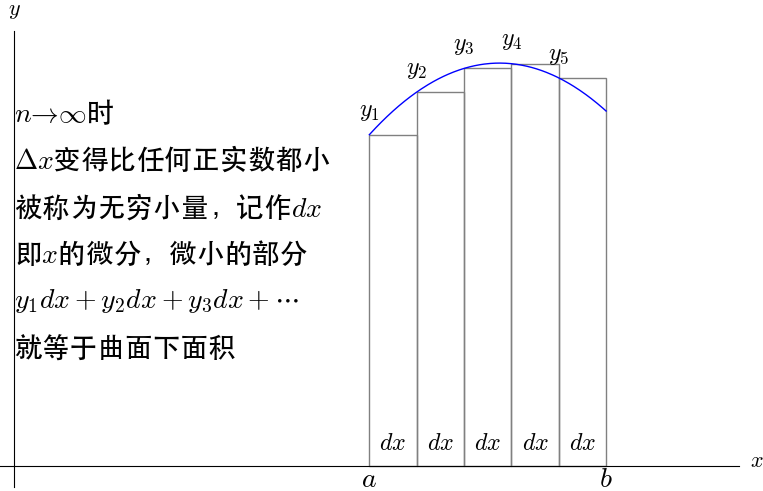1.3 导数的古典定义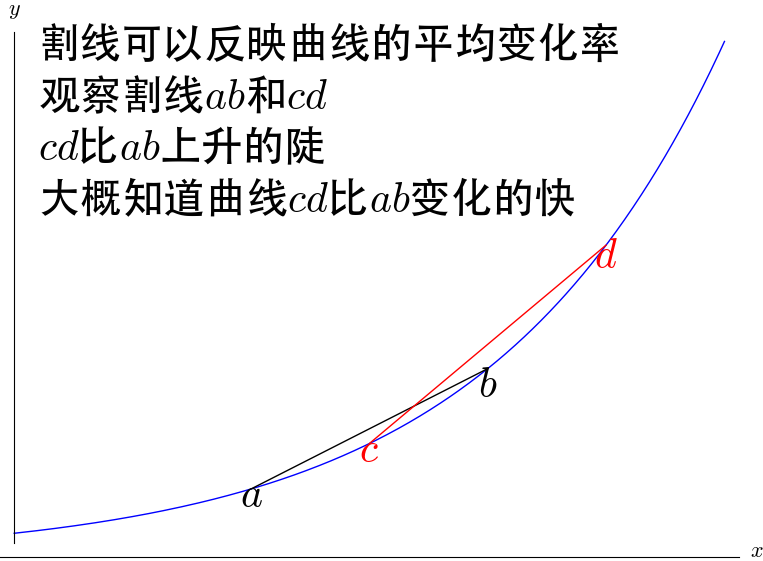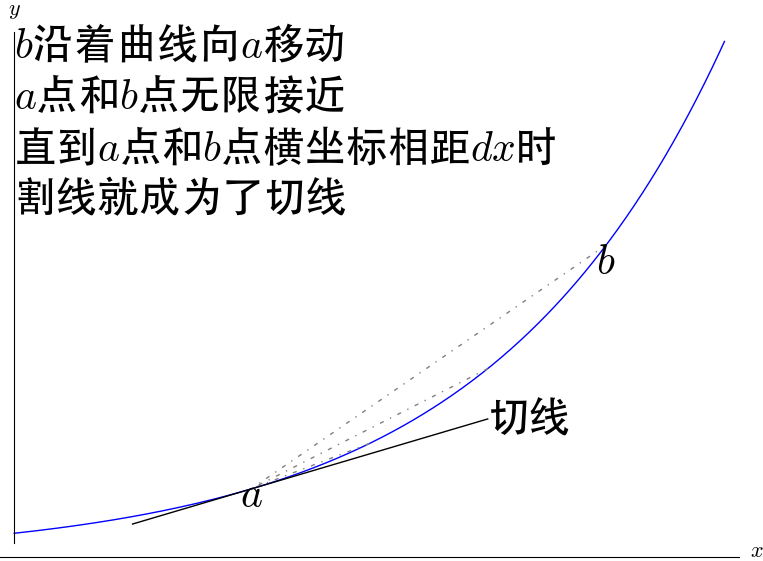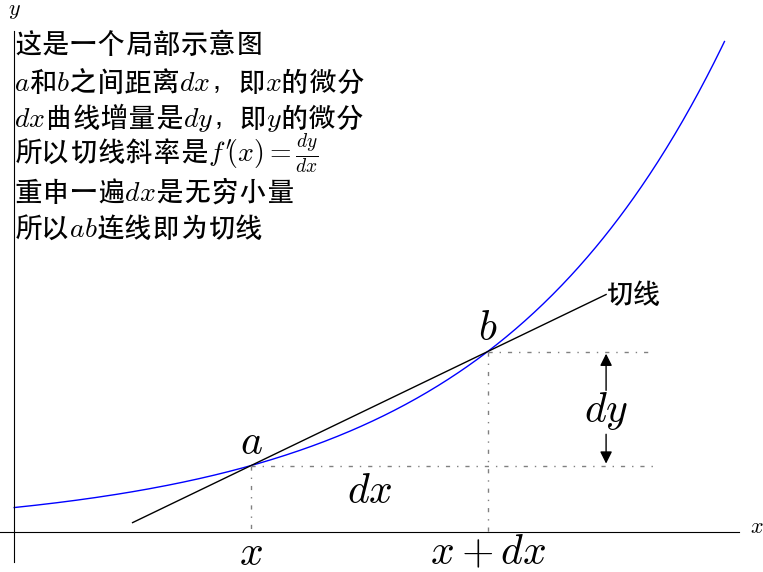1.4 无穷小量导致的麻烦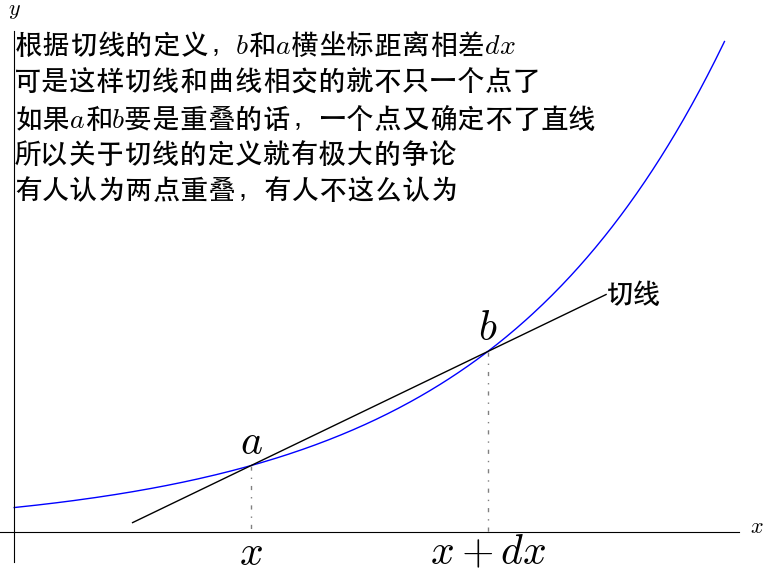\begin{align*} \frac{d}{dx}(x^2) & = \frac{f(x+dx)-f(x)}{dx} \\ & = \frac{(x+dx)^2-x^2}{dx} \\ & = \frac{x^2+2xdx+dx^2-x^2}{dx} \\ & = \frac{2xdx+dx^2}{dx} \\ & = 2x+dx \\ & = 2x \end{align*}

1.5 对于古典微积分的总结

• 切线：通过无穷小量定义了切线
• 导数：导数就是切线的斜率
• 微分：微分是微小的增量，即无穷小量

2 基于极限重建微积分

2.1 极限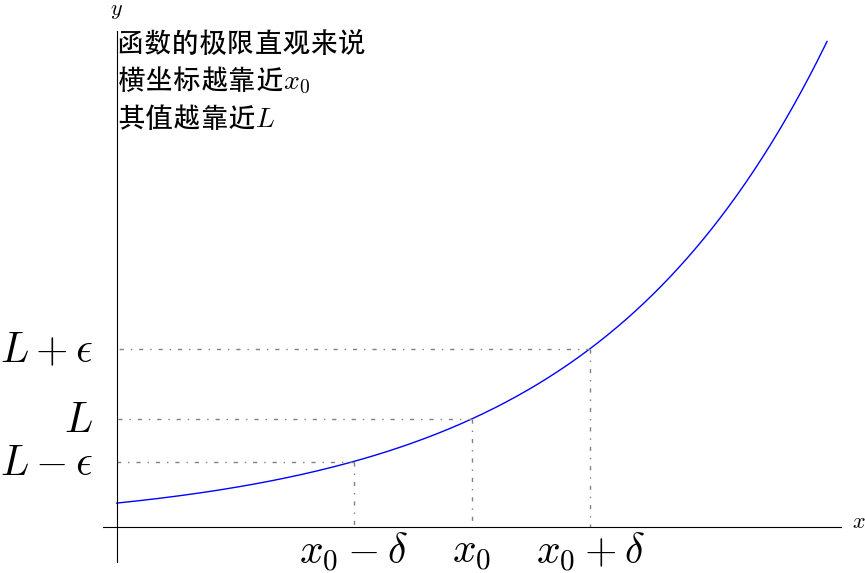2.2 导数的极限定义

\begin{align*} \displaystyle f'(x_0)& =\frac{dy}{dx}\\ & =\lim _{\Delta x \to 0}\frac{\Delta y}{\Delta x}\\ & =\lim _{\Delta x \to 0}\frac{f(x_0+\Delta x)-f(x_0)}{\Delta x} \end{align*}

\begin{align*} \displaystyle \lim _{\Delta x\to 0}\frac{\Delta y}{\Delta x}=f'(x_0) & \implies \lim _{\Delta x\to 0}\frac{\Delta y}{\Delta x}-f'(x_0)=0\\ & \implies \frac{\Delta y}{\Delta x}-f'(x_0)=a,\lim _{\Delta x \to 0}a=0\\ & \implies \Delta y=f'(x_0)\Delta x+a\Delta x \end{align*}

$\Delta y=f'(x_0)\Delta x+a\Delta x$可以得出，$\Delta y$由两部分组成，通过图来观察一下几何意义：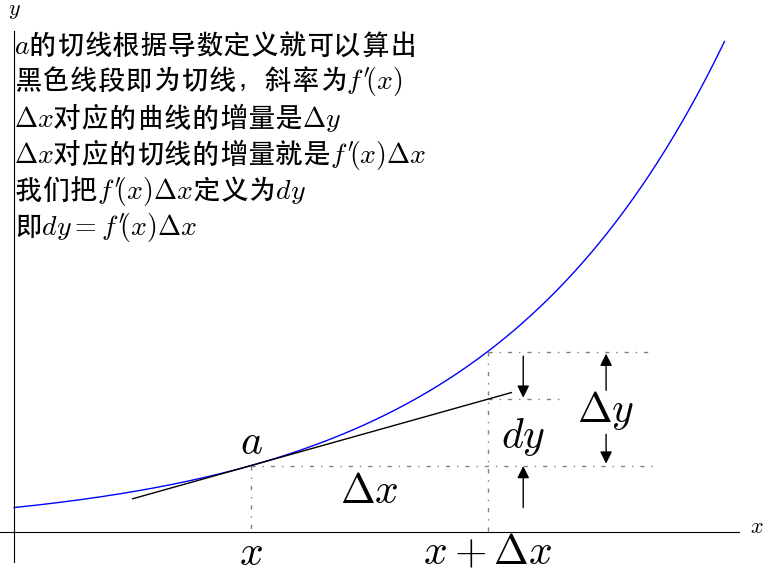$dy=f'(x)\Delta x$，这是$dy$的定义。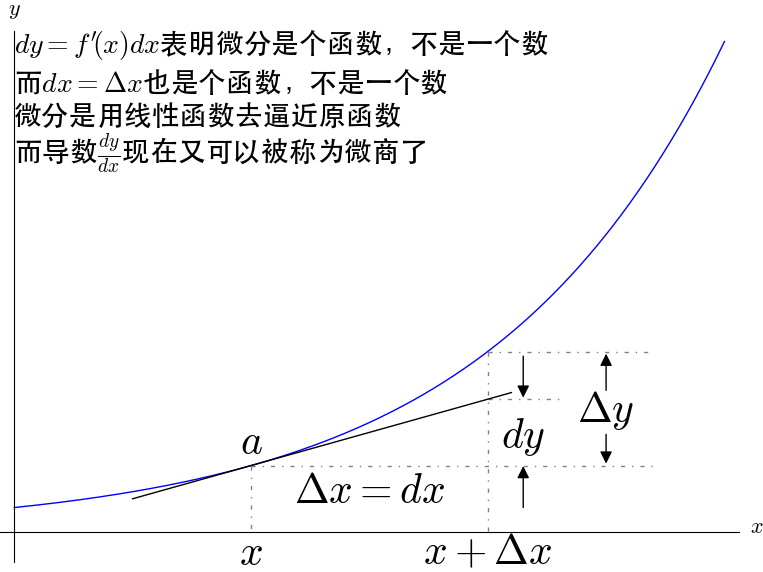2.3 对于极限微积分的总结

• 导数：被定义为一个极限，其意义就是变化率
• 微分：是一个线性函数，其意义就是变化的具体数值
• 切线：有了导数之后就可以被确定下来了

3 疑问的解答

3.1 古典微积分与极限微积分的对比

• 古典微积分是先定义微分再定义导数，极限微积分是先定义导数再定义微分。
• 古典微积分的导数是基于无穷小量定义的，极限微积分的导数是基于极限定义的。
• 古典微积分的微分是无穷小量，极限微积分的微分是一个线性函数。
• 古典微积分的定积分是求无穷小矩形面积的和，极限微积分的定积分是求黎曼和。
• 古典微积分的切线是可以画出来的，极限微积分的切线是算出来的。
• 古典微积分的建立过程很直观，极限微积分的建立过程更抽象。

3.2 疑问的解答

• $\frac{dy}{dx}=\frac{dy}{du}\frac{du}{dx}$，在古典微积分中可以理解为消去，但是在极限微积分中我们应该认识到，这两个$du$实际上是不同的函数（第一个$du$是$u$的函数，第二个$du$是$x$的函数，其实$d$应该是一个二元操作符，第一个$du$可以写成$d(u,u)$，第二个$du$可以写成$d(u,x)$，这样一看就知道不是一个东西了）。
• $\int _ a^ b \frac{dy}{dx}dx$古典微积分中，$dx$确实表明是无穷多个矩形的底边，消去也是合理的，而极限微积分中，$\int _ a^ b dx$是求黎曼和，我们可以把$\int _ a^ b$当作左括号，$dx$当作右括号，就好比$(2+6)=8$，计算完毕之后，括号自然就消失了。
• $d(uv)=(u+du)(v+dv)-uv=udv+vdu+dudv$在古典微积分中这么计算没有错误，只是$dudv$的消去也是不严谨的，而极限微积分中应该重新用极限的方法进行证明，这里不再列出。

3.3 古典微积分的用处

4 无穷小量的逆袭